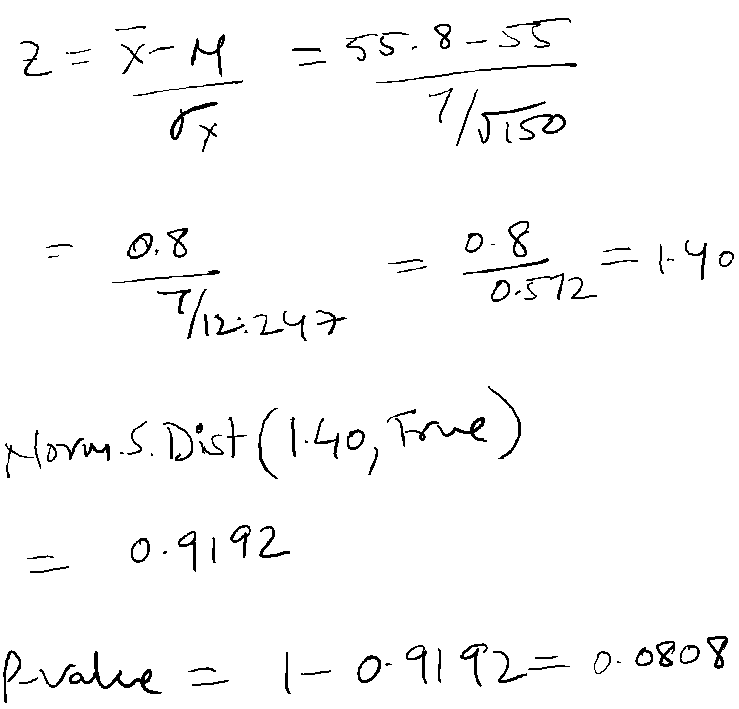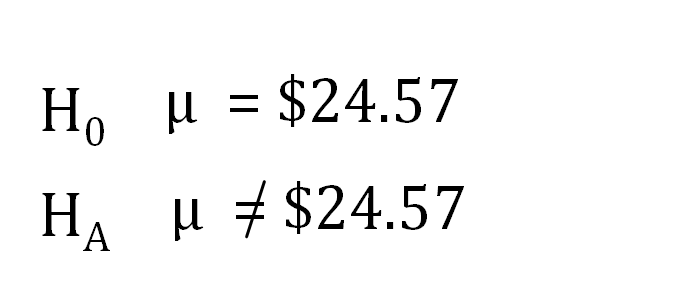Nishant Malhotra Founder of Middle Road OPC Pvt Ltd & The middle Road platform talks about the startup

### Ahoy Ahoy-Ahoy!

Welcome to the Kickass platform enabling social change & impact

# Statistics Module 4: Questions

You are here:
• Statistics Module 4: Questions

Solved questions on hypothesis testing for Known population standard deviation

1. Consider the following hypotheses test.a. For a sample size of 189 and a sample mean of  55.8, compute the value of test statistic if the population standard deviation is 7.

b. Is this a single or double tailed test?

c. What’s is the p-value and use α =0.05 to derive your conclusion.

d. Looking at the critical value, how can you derive the conclusion.

e. Do you think you need to consider a 1% level of significance test here?The p-value is 0.0808 > 0.05, so the null hypotheses cannot be rejected. The population mean is greater or equal to 55.

In a single tailed test at α =0.05, the Z value can be calculated using Norm.inv for 0.05.

Norm.inv (0.05, 0,1) = 1.65

The Z value is 1.65. Since the sample Z value is 1.40 which is less than 1.65, the null cannot be rejected.

No. 1%  level of significance test does not matter since the p-value is greater than α =0.05.

2. The mean hourly wage of employees in goods producing industries is currently \$24.57 (Bureau of Labor Stats website April 12, 2012), Suppose we take a sample of employees from the manufacturing industry to see if the mean hourly wage differs from the reported mean of \$24.57 for the good producing industries. (Statistics for Business & Economics by Anderson | Sweeney | Williams | Camm | Cochran.)

a. State the null and alternative hypotheses we should use to test whether the population mean hourly wage in the manufacturing industry differs from the population mean hourly wage in the goods producing industries.

b. Suppose a sample of 30 employees from the manufacturing industry showed a sample mean of \$23.89 per hour. Assume a population S.D of \$2.40 per hour and compute the p value.

c. At α =0.05, what is your conclusion?

d. Repeat the proceeding hypothesis test using the critical value approach?µ gs = mean hourly wage for employees in the goods producing industry = \$24.57 per hour

µ ms = mean hourly wage for employees in the manufacturing industry = \$23.89 per hourMean hourly wage of employees in goods producing industries does not differ from the mean hourly wage of employees in manufacturing industry.

p-value > 0.05, we cannot reject the null

Critical value is -1.96 and 1.96.  -1.55 > -1.96 and 1.55 < 1.96 so we cannot reject the null.

3. In a country, the mean number of hours for watching television per week is 12.5 hours.  However recently, a study conducted in the northern part of this country with a sample size of 256 people found the mean hours per week for watching television to be 13.15 hrs. Assume population standard deviation to be 2.5 hrs.

• Design a hypothesis test for the above problem to test whether the claim that the mean hours per week for watching the television is more in the northern part than the national average? Test at 0.05 level of significance.
• Test at α =0.01. What is your conclusion?

Check out the video for the solution for the above problem on hypothesis testing. Check out the tutorial on hypothesis testing for unknown population standard deviation  using t-distribution.